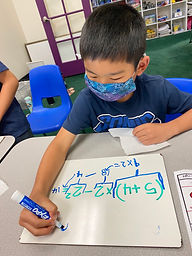Ms. Brianne

Target 1​

Lesson Type:

Continuation

Number Operation

:

Computation

Solve multi-step problems within 100 (addition, subtraction, multiplication, and division).

1:

Solve problems that have more than one operation.

2:

Understand that the presence of parenthesis in an arithmetic equation signals for this part of the problem to be solved first.

3:

Understand when solving a problem that has both addition and subtraction operations that solving starts on the left and continues to the right.

3rd

Vocabulary:

PEMDAS, Order of Operations

Activities:

• Students were introduced to parentheses. They learned that if there is a set of parenthesis, they must solve that part of the equation first.
• Students continued to practice following the order of operations to solve multi-step problems with multiple operations.
• Students solved multi-step problems and utilized their answers to color in a picture following a color key.Home Exploration

Guiding Questions:Absent Students:

Max

Target 2

:

1:

Imagine an object being manipulated in space (rotated, folded, cut, etc.)

Vocabulary:

Coordinates, 2D, 3D, Cut, Fold

Activities:

• Students reviewed coordinates, and plotted a set of given coordinates on a grid to create the 2D diagram.
• Students used spatial reasoning to predict the 3D structure that would be created when the 2D diagram was cut and folded.Home Exploration

Guiding Questions:Target 3

:

Vocabulary:

Activities:Home Exploration